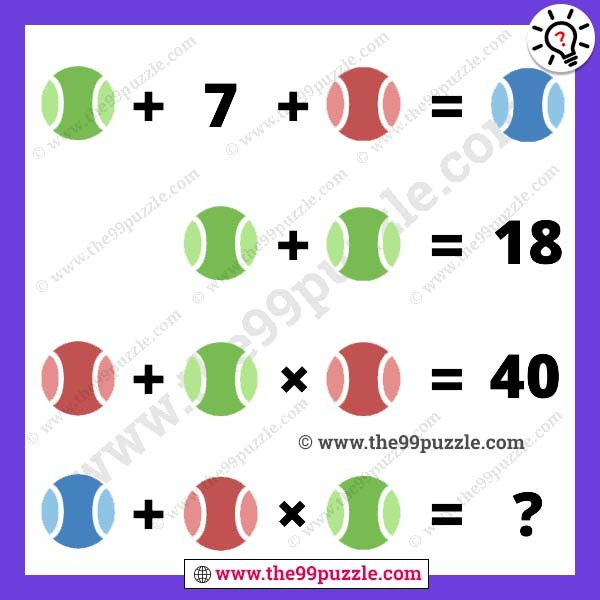# Ball picture mind cracking puzzle with answer – Puzz244

Solve the ball picture mind cracking puzzle. Most puzzlers fail to solve this picture puzzle. This math picture is more tricky than other picture puzzles. If you practice more math equation puzzles, find this website and try to solve all these puzzles. To learn and solve quickly math tricks by the practice of these puzzles math. In this picture, you see three different types of balls are in the equation. If you are smart in puzzles you can solve this answer.###### Explanation:

Green Ball + 7 + Red Ball = Blue Ball

Green Ball + Green Ball = 18

Red Ball + Green Ball × Red Ball = 40

Blue Ball + Red Ball × Green Ball = ?

Green Ball = 9

Red Ball = 4

Blue Ball = 20

9 + 7 + 4 = 20

9 + 9 = 18

4 + 9 × 4 = 40

20 + 4 × 9 = 56